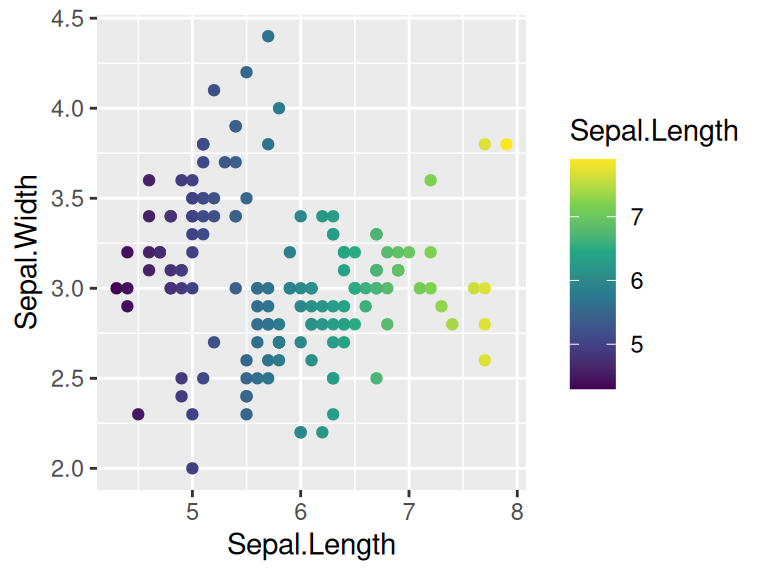# Chapter 15 Colors

When evaluating the power and efficiency of a plot, color is always a key factor that sometimes speaks a language even louder than words. So in this chapter, you will be introduced with several widely-applied color schemes and get to know how to use proper colors to make better plots based on different features of your data.

## 15.1 RColorBrewer

`RColorBrewer` is an R package having built-in sensible color schemes ready-to-use for figures. Colors are grouped into three types: sequential, diverging, and qualitative.

Sequential – Light colours for low data, dark for high data

Qualitative(for categorical data) – Colours designed to give maximum visual difference between categories so great for non-ordered categorical data

Diverging – Light colours for mid-range data, low and high use dark colours, great to seperate two extremes

``````library(RColorBrewer)
display.brewer.all() ``````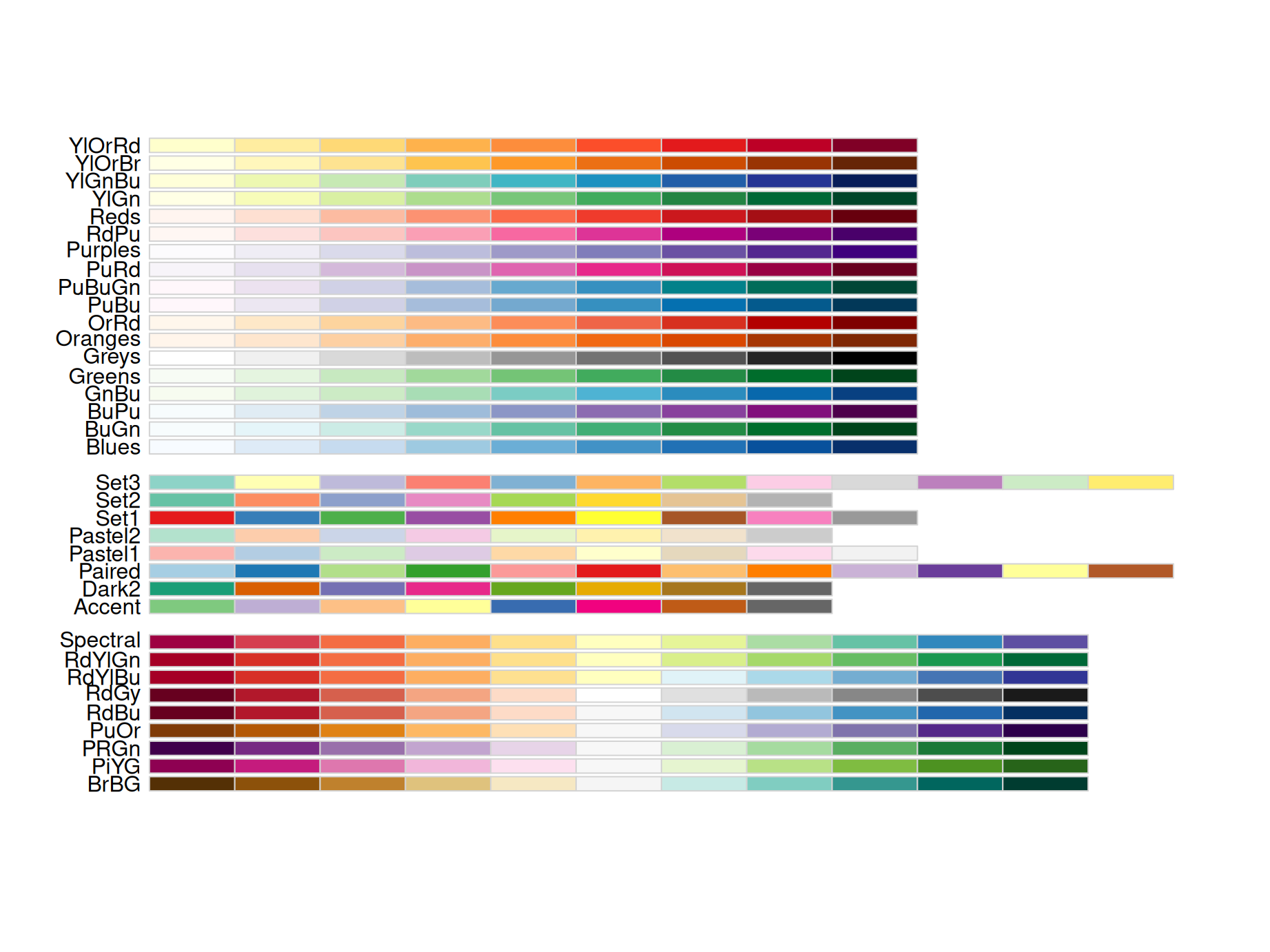Here is an example of plotting categorical data using `Dark2` pallets under qualitative group of RColorBrewer:

``````library(ggplot2)
ggplot(iris, aes(Petal.Length, Sepal.Length, colour = Species)) +
geom_point() +
scale_colour_brewer(palette = "Dark2")``````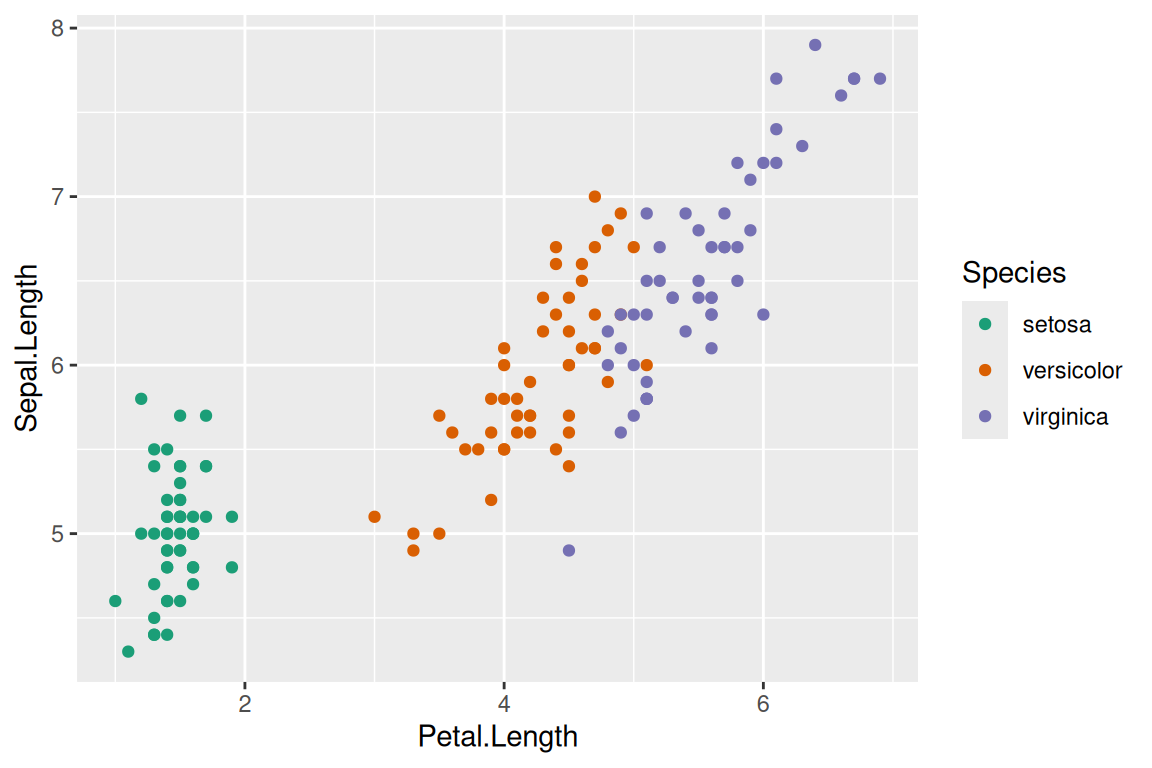Also, you can create your own sequential pallets.

``````ggplot(faithfuld, aes(waiting, eruptions, fill = density)) +
geom_raster() +
scale_fill_gradient(low = "white", high = "red")``````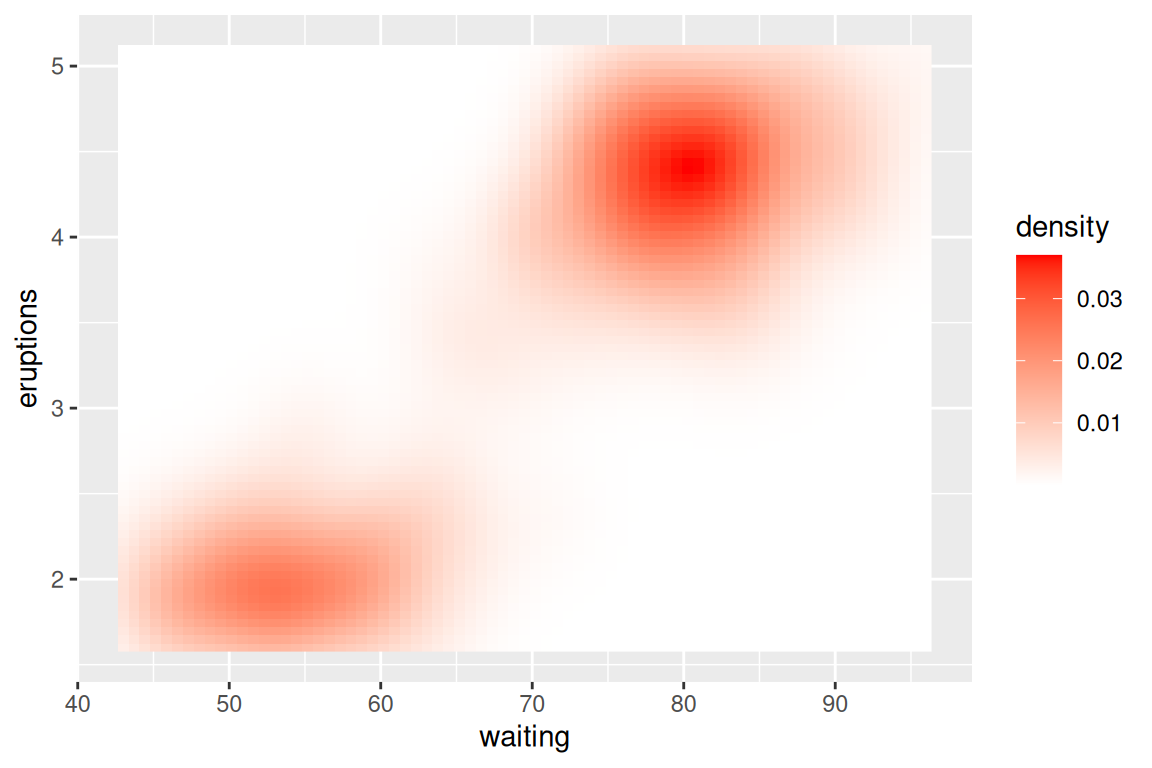Or diverging pallets:

``````ggplot(faithfuld, aes(waiting, eruptions, fill = density)) +
geom_raster() +
scale_fill_gradient2(low = "grey", mid = "white", high = "red",midpoint = .02)``````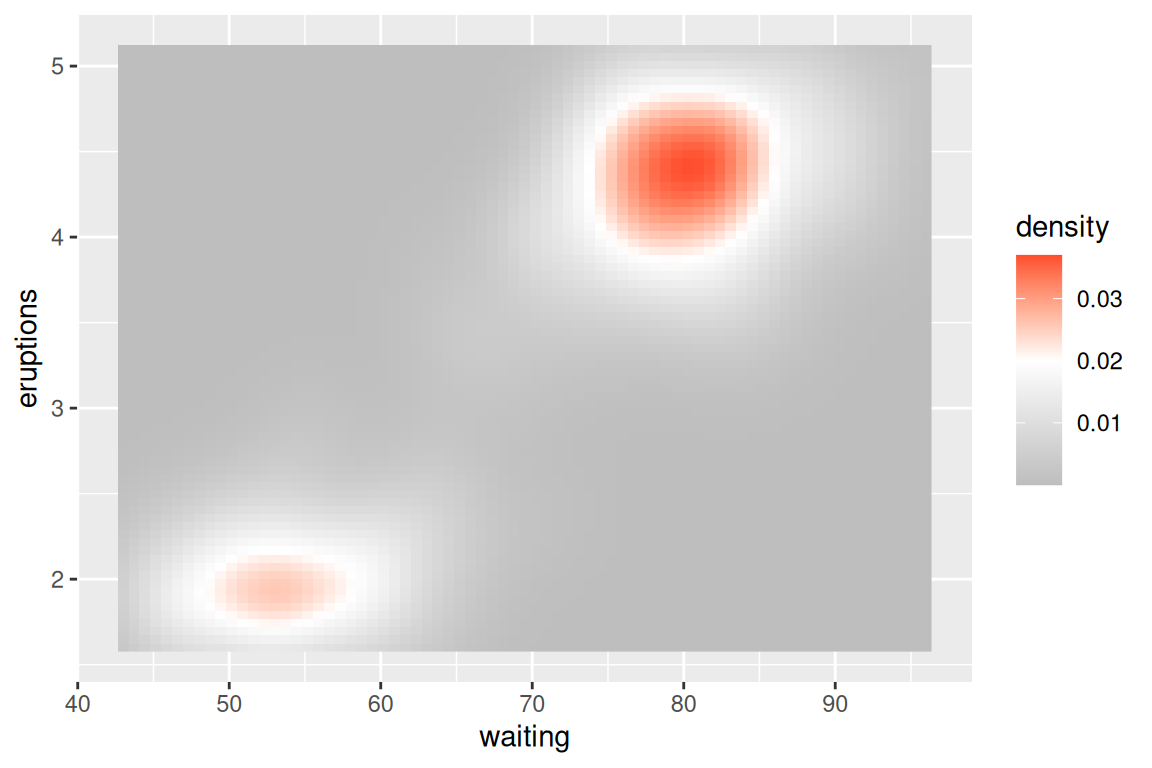For discrete data, using `scale_colour_manual` is a good choice. For discrete ordinal data, we can use another package (such as vcd)

``````ggplot(mtcars, aes(mpg, wt)) +
geom_point(aes(colour = factor(cyl))) +
scale_colour_manual(values = c("red", "yellow", "blue"))``````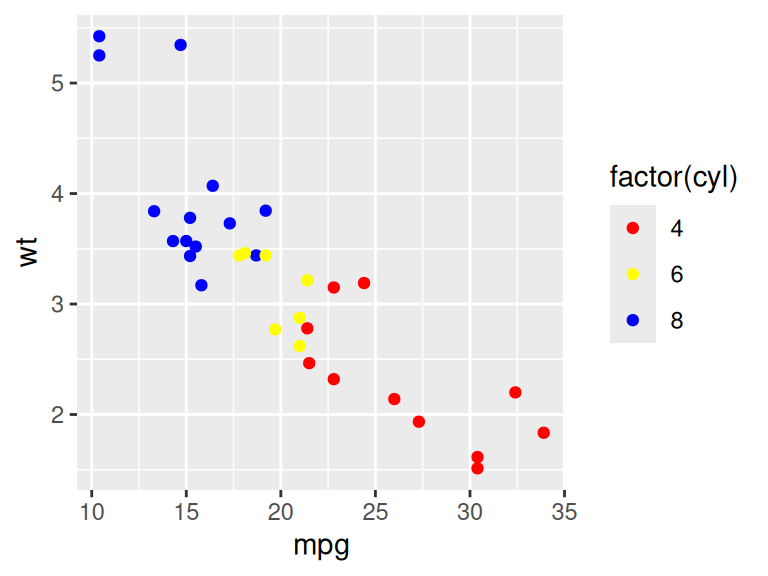``````colors<-brewer.pal(5,'Blues')
barplot(1:5, col=colors)``````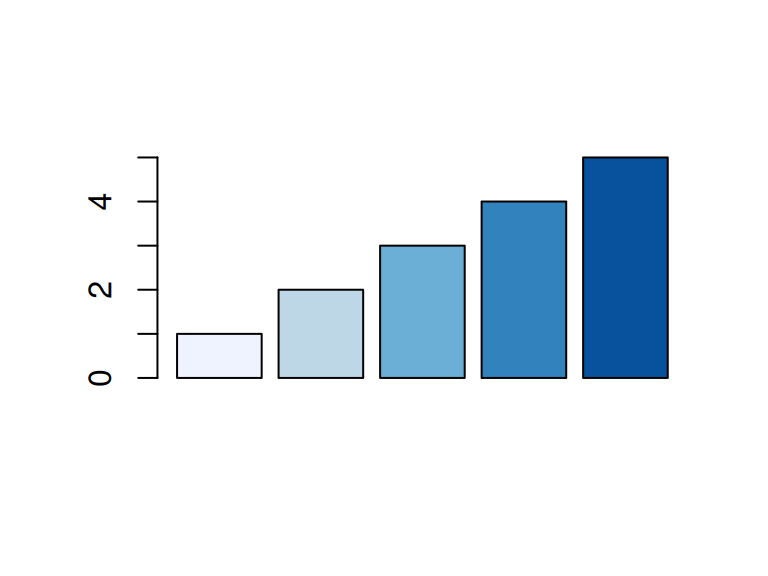## 15.2 Perceptually uniform color spaces: Viridis

The `viridis` R package provides four palettes for use in R which are pretty, perceptually uniform and easy to read by those with colorblindness.

The package contains eight color scales: `viridis`, the primary choice, and five alternatives with similar properties - `magma`, `plasma`, `inferno`, `civids`, `mako`, and `rocket` -, and a rainbow color map - `turbo`.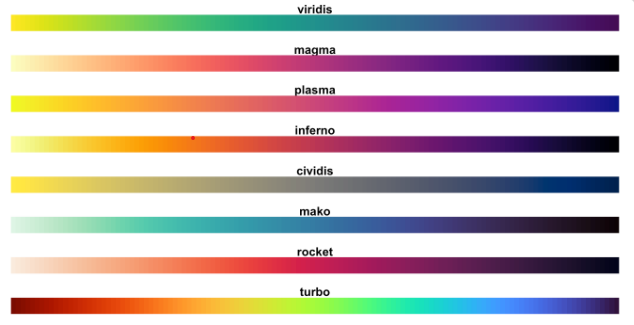Perceived differences are proportional to scalar differences when using `viridis`. The following example shows `viridis`on continuous data using `scale_color_viridis_c`, use `scale_color_viridis_d()` for discrete data

``````library("viridis")
ggplot(iris, aes(Sepal.Length, Sepal.Width))+
geom_point(aes(color = Sepal.Length)) +
scale_color_viridis_c()``````